# On double-diffusive convection and cross diffusion effects on a horizontal wavy surface in a porous medium

## Abstract

An analysis of double diffusive convection induced by a uniformly heated and salted horizontal wavy surface in a porous medium is presented. The wavy surface is first transformed into a smooth surface via a suitable coordinate transformation and the transformed nonsimilar coupled nonlinear parabolic equations are solved using the Keller box method. The local and average Nusselt and Sherwood numbers are given as functions of the streamwise coordinate and the effects of various physical parameters are discussed in detail. The effects of the Lewis number, buoyancy ratio, and wavy geometry on the dynamics of the flow are studied. It was found, among other observations, that the combined effect of Dufour and Soret parameters is to reduce both heat and mass transfer.

MSC:34B15, 65N30, 76M20.

## 1 Introduction

The study of double-diffusive convection has received considerable attention during the last several decades because of its occurrence in a wide range of natural and technological settings. Double-diffusive convection is an important fluid dynamic phenomenon that involves motions driven by two different density gradients diffusing at different rates (Mojtabi and Charrier-Mojtabi ). A common example of double diffusive convection is seen in oceanography, where heat and salt concentrations exist with different gradients and diffuse at differing rates. Double diffusive convection manifests in the form of “salt-fingers” (see Stern [2, 3]) which are observable in laboratory settings. The input of cold fresh-water from an iceberg can affect both of these variables. Double-diffusive convection has also been cited as being important in the modeling of solar ponds (Akbarzadeh and Manins ) and magma chambers (Huppert and Sparks , Fernando and Brandt ). Double-diffusive free convection is also seen in sea-wind formations, where upward convection is also modified by Coriolis forces. This is of particular interest in oceans where the Earth’s rotation plays a dominant role in many of the motions observed.

In engineering applications, double diffusive convection is commonly visualized in the formation of microstructures during the cooling of molten metals, and fluid flows around shrouded heat-dissipation fins. Typical technological motivations for the study of double-diffusive convection range from such diverse fields as the migration of moisture through air contained in fibrous insulations, grain storage systems, the dispersion of contaminants through water-saturated soil, crystal growth, solidification of binary mixtures, and the underground disposal of nuclear wastes. Other important applications can be found the fields of geophysical sciences and electrochemistry. A comprehensive review of the literature concerning natural convection in fluid-saturated porous media may be found in the books by Ingham and Pop [7, 8], Nield and Bejan , Vafai [10, 11], and Vadasz .

Most free convection studies mainly focus on the cases where the thermal boundary conditions allow the use of similarity transformations to reduce the governing boundary layer equations to a system of ordinary differential equations which can be handled either analytically or numerically. The existence of self-similar solutions point to the fact that, in general, the heated surface must have a plane geometry. In reality, the heated surfaces need not always be planar. Surfaces are sometimes deliberately roughened to achieve enhanced heat transport. Heat transfer devices like flat-plate solar collectors and flat-plate condensers in refrigerators possess a nonuniform surface. Further, in cavity wall insulating systems and grain storage systems, one can witness pronounced surface roughness. Yao  and Moulic and Yao [14, 15] were the first to include the effects of surface nonuniformities on the free convection thermal boundary layer flow of a Newtonian fluid. Rees and Pop [16, 17] investigated parallel thermal boundary layers due to natural convection induced by a vertical surface with sinusoidal undulations embedded in a in porous medium. Subsequent studies by Rees and Pop [18, 19] considered the effect of surface waves on the convection due to either horizontal or vertical wavy surfaces embedded in a porous medium. In the case of horizontal wavy surface, Rees and Pop  found that the amplitude of the surface waves must be within an $O\left({\mathit{Ra}}^{-1/3}\right)$ range in order to balance direct and indirect buoyancy forces. They showed that for wall amplitudes greater than about $0.95{\mathit{Ra}}^{-1/3}$, the flow separates with one or more regions of reverse flow. Rees and Pop  obtained similarity solutions for the case of longitudinal surfaces waves with (i) a prescribed power-law temperature is set on the surface, and (ii) a prescribed power-law heat flux.

Cheng , in a series of papers investigated free convection due to vertical/inclined wavy surfaces in porous media. Cheng  studied natural convection, heat and mass transfer near a vertical wavy surface with constant wall temperature and concentration in a porous medium. He showed that the average Nusselt and Sherwood numbers for a sinusoidal wavy surface are constantly smaller than the corresponding results for a flat plate. This work was generalized in Cheng  to a non-Darcy model for natural convection heat and mass transfer from a vertical wavy surface in a porous media. Cheng  considered a non-Newtonian power law liquid to study combined heat and mass transfer in free convection flow due to a vertical wavy surface in a porous medium with thermal and mass stratifications. It was found that an increase in the power-law index, the thermal stratification parameter, or the concentration stratification parameter leads to a smaller fluctuation of the local Nusselt and Sherwood numbers with the streamwise coordinate. Double diffusive natural convection along an inclined wavy surface in a porous medium was investigated by Cheng  and showed that the inclination angle enhanced the total heat and mass transfer rates.

Pop and Na  studied natural convection due to a frustum of a wavy cone in a porous medium and discussed the effects of geometry of a wavy cone on the heat transfer. Cheng  studied the natural convection heat and mass transfer near a wavy cone with constant wall temperature and concentration in a porous medium. Using a cubic spline method of solution, he showed that the buoyancy ratio leads to higher heat and mass transports while the diffusivity ratio (Lewis number) reduces heat while increasing mass transports. Cheng  obtained nonsimilar solutions for double diffusive convection near a frustum of a wavy cone in porous media and showed that the average Nusselt and Sherwood numbers for the wavy cone are smaller than the corresponding smooth cone. Double-diffusive natural convection along a vertical truncated wavy cone in non-Newtonian fluid saturated porous media with thermal and mass stratification has been investigated by Cheng . In this study, he showed that the power-law index leads to smaller fluctuations in the local Nusselt and Sherwood numbers and increasing the thermal and concentration stratification parameters reduce the heat and mass transfer rates.

An energy flux is often generated by both temperature and solute gradients leading to Dufour or diffusion-thermo effect and Soret or thermal-diffusion effects. Both effects have been extensively studied in gases, while the Soret effect has been studied both theoretically and experimentally in liquids; see Mortimer and Eyring . It is generally accepted that the Dufour and the Soret effects are small compared to the effects described by Fourier and Fick’s laws (Mojtabi and Charrier-Mojtabi ) and can therefore be neglected in many heat and mass-transfer processes. However, it has been shown in a number of studies that there are exceptions in areas such as in geosciences where Dufour and Soret effects are significant and cannot be ignored; see for instance Kafoussias and Williams , Awad et al. , and the references therein.

Mortimer and Eyring  used an elementary transition state approach to obtain a simple model for Soret and Dufour effects in thermodynamically ideal mixtures of substances with molecules of nearly equal size. In their model, the flow of heat in the Dufour effect was identified as the transport of the enthalpy change of activation as molecules diffuse. The results were found to fit the Onsager reciprocal relationship, Onsager . Shariful et al.  investigated the Dufour and Soret effects on steady combined free-forced convective and mass transfer flow past a semi-infinite vertical flat plate of hydrogen-air mixtures. They used the fourth-order Runge-Kutta method to solve the governing equations of motion. Their study showed that the Dufour and Soret effects should not be neglected. Shateyi et al.  investigated the effects of diffusion-thermo and thermal-diffusion on MHD fluid flow over a permeable vertical plate in the presence of radiation and hall current. Recently, Narayana et al.  and Malashetty and Biradar  have investigated the cross diffusion effects on the onset of double diffusive convection in a binary Maxwell fluid saturated porous medium. Other related recent studies on the effects of Soret and Dufour parameters include those by Makinde .

In this paper, we extend the work by Rees and Pop  to include the solute diffusion in natural convection induced by a horizontal wavy surface profile in a porous medium. The wall temperature and concentrations are assumed to be constants and the amplitude of the wave is assumed to be small enough so as not to engender any regions of reverse flow (these issues are dealt in detail by Rees and Pop ). Assuming the Rayleigh number Ra to be very large, the boundary layer approximation is invoked leading to a set of nonsimilar parabolic partial differential equations whose solution is obtained using the Keller box method (see, Keller , Keller and Cebeci ).

## 2 Mathematical formulation

Consider the problem of double-diffusive convection in a fluid around a horizontal surface with transverse sinusoidal undulations embedded in a porous medium. Figure 1 shows the schematic sketch of the problem. The wavy surface profile is described by

$y={\sigma }^{\ast }\left(x\right)={a}^{\ast }sin\left(\pi x/l-\phi \right),$
(1)

where ${a}^{\ast }$ is the amplitude of the surface wave, l is the characteristic length of the wave, and φ is the phase of the wave.

The temperature and the solute concentrations at the surface are assumed to be constants ${T}_{w}$ and ${C}_{w}$ which are greater than the ambient values ${T}_{\mathrm{\infty }}$ and ${C}_{\mathrm{\infty }}$, respectively. We also assume a steady convective flow with the properties of the fluid and porous medium to be constant except for the buoyancy term. Following the Boussinesq approximation, the governing continuity, momentum, heat, and solute concentration equations can be written in the form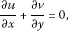(2)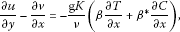(3)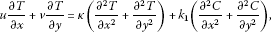(4)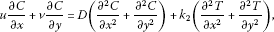(5)

subject to boundary conditions

(6)

Here, g is the acceleration due to gravity, K is permeability of the porous medium, ν is the kinematic viscosity, β is the coefficient of thermal expansion, ${\beta }^{\ast }$ is the coefficient of solutal expansion, κ is the thermal diffusivity, D is the solutal diffusivity, ${k}_{1}$ and ${k}_{2}$ are the coefficients of cross diffusion. We now use the following nondimensional variables:

$\begin{array}{r}\left(X,Y,R,\sigma ,a\right)=\frac{\left(x,y,r,{\sigma }^{\ast },{a}^{\ast }\right)}{l},\phantom{\rule{2em}{0ex}}\left(U,V\right)=\frac{\left(u,v\right)}{\kappa /l},\\ \overline{T}=\frac{T-{T}_{\mathrm{\infty }}}{{T}_{w}-{T}_{\mathrm{\infty }}}\phantom{\rule{1em}{0ex}}\text{and}\phantom{\rule{1em}{0ex}}\overline{C}=\frac{C-{C}_{\mathrm{\infty }}}{{C}_{w}-{C}_{\mathrm{\infty }}}.\end{array}$
(7)

The governing equations (2)-(5) take the form: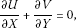(8)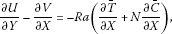(9)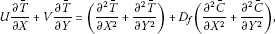(10)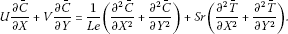(11)

The dimensionless parameters appearing in the above set of equations are the Rayleigh number Ra, the buoyancy ratio N, Dufour number ${D}_{f}$, the Soret number Sr, and the Lewis number Le defined as follows:

$\begin{array}{r}\mathit{Ra}=\frac{\mathrm{g}\beta K\left({T}_{w}-{T}_{\mathrm{\infty }}\right)l}{\nu \kappa },\phantom{\rule{2em}{0ex}}N=\frac{{\beta }^{\ast }}{\beta }\left(\frac{{C}_{w}-{C}_{\mathrm{\infty }}}{{T}_{w}-{T}_{\mathrm{\infty }}}\right),\phantom{\rule{2em}{0ex}}{D}_{f}=\frac{{k}_{1}}{\kappa }\left(\frac{{C}_{w}-{C}_{\mathrm{\infty }}}{{T}_{w}-{T}_{\mathrm{\infty }}}\right),\\ Sr=\frac{{k}_{2}}{\kappa }\left(\frac{{T}_{w}-{T}_{\mathrm{\infty }}}{{C}_{w}-{C}_{\mathrm{\infty }}}\right),\phantom{\rule{2em}{0ex}}\mathit{Le}=\frac{\kappa }{D}.\end{array}$
(12)

The boundary conditions in (6) take the following nondimensional form:

(13)

Introducing the stream function $\psi \left(X,Y\right)$, such that

$U=\frac{\partial \psi }{\partial Y},\phantom{\rule{2em}{0ex}}V=-\frac{\partial \psi }{\partial X},$
(14)

the continuity equation (8) is satisfied automatically and Eqs. (9)-(11) can be written in the following form: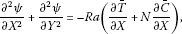(15)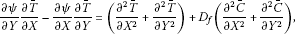(16)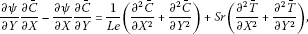(17)

with the boundary conditions

(18)

The effect of the wavy surface and the usual boundary layer scalings are incorporated into the governing equations (15)-(17) using the transformations,

$X=\xi ,\phantom{\rule{2em}{0ex}}Y={\mathit{Ra}}^{-1/3}{\xi }^{2/3}\eta +asin\left(\pi \xi -\phi \right)$
(19)

along with the substitutions

$\psi ={\mathit{Ra}}^{1/3}{\xi }^{1/3}f\left(\xi ,\eta \right),\phantom{\rule{2em}{0ex}}\overline{T}=\theta \left(\xi ,\eta \right),\phantom{\rule{2em}{0ex}}\overline{C}=\varphi \left(\xi ,\eta \right)\phantom{\rule{1em}{0ex}}\text{and}\phantom{\rule{1em}{0ex}}a={\mathit{Ra}}^{-1/3}\alpha .$
(20)

Equations (19) transform the wavy surface to a smooth surface in the physical configuration. Substituting (19) and (20) into Eqs. (15)-(18) and letting $\mathit{Ra}\to \mathrm{\infty }$, we obtain the following boundary layer equations:(21)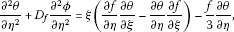(22)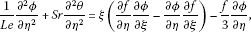(23)

subject to the boundary conditions

$\begin{array}{r}f\left(\xi ,0\right)=0,\phantom{\rule{2em}{0ex}}\theta \left(\xi ,0\right)=1,\phantom{\rule{2em}{0ex}}\varphi \left(\xi ,0\right)=1,\\ \frac{\partial f}{\partial \eta }\left(\xi ,\mathrm{\infty }\right)\to 0,\phantom{\rule{2em}{0ex}}\theta \left(\xi ,\mathrm{\infty }\right)\to 0,\phantom{\rule{2em}{0ex}}\varphi \left(\xi ,\mathrm{\infty }\right)\to 0.\end{array}$
(24)

The associated local Nusselt and Sherwood numbers

$\frac{{\mathit{Nu}}_{x}}{{\mathit{Ra}}^{1/3}}=-{\xi }^{1/3}{\theta }_{\eta }\left(\xi ,0\right),\phantom{\rule{2em}{0ex}}\frac{{\mathit{Sh}}_{x}}{{\mathit{Ra}}^{1/3}}=-{\xi }^{1/3}{\varphi }_{\eta }\left(\xi ,0\right).$
(25)

The mean Nusselt and Sherwood numbers from the leading edge to the free stream position x are given by

$\frac{{\mathit{Nu}}_{m}}{{\mathit{Ra}}^{1/3}}=-{\int }_{0}^{\xi }{\xi }^{-2/3}{\theta }_{\eta }\left(\xi ,0\right)\phantom{\rule{0.2em}{0ex}}d\xi ,\phantom{\rule{2em}{0ex}}\frac{{\mathit{Sh}}_{m}}{{\mathit{Ra}}^{1/3}}=-{\int }_{0}^{\xi }{\xi }^{-2/3}{\theta }_{\eta }\left(\xi ,0\right)\phantom{\rule{0.2em}{0ex}}d\xi .$
(26)

## 3 Solution procedure

The nonsimilar parabolic partial differential equations that govern the dynamics of the fluid flow were solved using the Keller box implicit finite difference method. This section gives details of the Keller box scheme used to solve the coupled nonlinear parabolic equations (21)-(23). We present the implementation of the Keller box method for a problem that involves coupling, nonlinearity, variable coefficients. The method consists of four main steps; decomposition, discretization, linearization, and the solution of the linearization difference equation.

The decomposition of Eqs. (21)-(23) into first-order equations is achieved by setting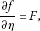(27)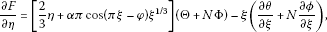(28)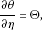(29)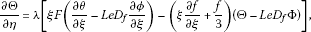(30)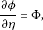(31)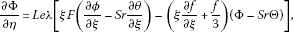(32)

where $\lambda ={\left(1-Sr{D}_{f}\right)}^{-1}$. The boundary conditions take the form

$\begin{array}{r}f\left(\xi ,0\right)=0,\phantom{\rule{2em}{0ex}}\theta \left(\xi ,0\right)=1,\phantom{\rule{2em}{0ex}}\varphi \left(\xi ,0\right)=1,\\ F\left(\xi ,\mathrm{\infty }\right)=0,\phantom{\rule{2em}{0ex}}\theta \left(\xi ,\mathrm{\infty }\right)=0,\phantom{\rule{2em}{0ex}}\varphi \left(\xi ,\mathrm{\infty }\right)=0.\end{array}$
(33)

In the discretization and formation of finite difference equations, the computational domain (ξ-η plane) is divided into a finite number of mesh points that form the corners of the rectangle shown in Figure 2(a). The net points are defined as

$\begin{array}{r}{\xi }_{0}=0,\phantom{\rule{2em}{0ex}}{\xi }_{i}={\xi }_{i-1}+{h}_{i},\phantom{\rule{1em}{0ex}}1\le i\le I,\\ {\eta }_{0}=0,\phantom{\rule{2em}{0ex}}{\eta }_{j}={\eta }_{j-1}+{k}_{j},\phantom{\rule{1em}{0ex}}1\le j\le J,{\eta }_{J}={\eta }_{\mathrm{\infty }},\end{array}$
(34)

where ${h}_{i}$ and ${k}_{j}$ are respectively the spacings in the ξ and η directions. The choice of net spacings is done manually in the present paper but can be generated automatically. A typical mesh rectangle over which Eqs. (27)-(32) are to be approximated is indicated in Figure 2(b). The equations are centered around one of the points marked by “×.”

A finer grid that points toward the surface ($\eta =0$) and a coarser grid that points away from the surface ($\eta \to {\eta }_{\mathrm{\infty }}$) are usually chosen for nonsimilar boundary layer flows, however, here we use uniform grid points in both directions. We fix ${\eta }_{\mathrm{\infty }}=10$ which is well outside the plane boundary layer surface. Since the boundary-layer equations have been formulated as a first-order system, all derivatives can be approximated by simple centered differences and two-point averages, using only values at the corners of the box. This type of differencing is as compact as possible and is, as we shall see, one of the most attractive features of the Box scheme. We use the notation ${\left[\cdot \right]}_{j}^{i}$ for any quantity represented midway between net points and this notion should not be confused with the tensorial one. Then, for any net quantity w we use the following finite difference approximations:

$\begin{array}{r}{\left[w\right]}_{j-\frac{1}{2}}^{i}=\frac{1}{2}\left({w}_{j}^{i}+{w}_{j-1}^{i}\right),\phantom{\rule{2em}{0ex}}{\left[\frac{\partial w}{\partial \eta }\right]}_{j-\frac{1}{2}}^{i}=\frac{1}{{k}_{j}}\left({w}_{j}^{i}-{w}_{j-1}^{i}\right),\\ {\left[\frac{\partial w}{\partial \xi }\right]}_{j-\frac{1}{2}}^{i-\frac{1}{2}}=\frac{1}{{h}_{i}}\left({\left[w\right]}_{j-\frac{1}{2}}^{i}-{\left[w\right]}_{j-\frac{1}{2}}^{i-1}\right),\phantom{\rule{2em}{0ex}}{\left[\frac{\partial w}{\partial \eta }\right]}_{j-\frac{1}{2}}^{i-\frac{1}{2}}=\frac{1}{2}\left({\left[\frac{\partial w}{\partial \eta }\right]}_{j-\frac{1}{2}}^{i}+{\left[\frac{\partial w}{\partial \eta }\right]}_{j-\frac{1}{2}}^{i-1}\right),\\ {\left[w\right]}_{j-\frac{1}{2}}^{i-\frac{1}{2}}=\frac{1}{2}\left({\left[w\right]}_{j-\frac{1}{2}}^{i}+{\left[w\right]}_{j-\frac{1}{2}}^{i-1}\right).\end{array}$
(35)

Using the box-scheme approximations (35), Eqs. (27)-(32) yield the following nonlinear finite difference equations: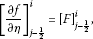(36)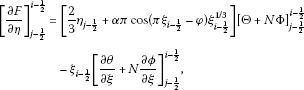(37)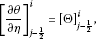(38)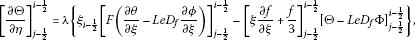(39)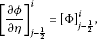(40)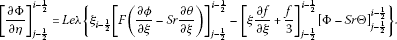(41)

Further manipulation yields the following equations: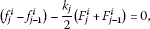(42)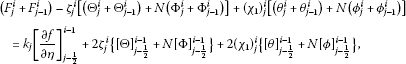(43)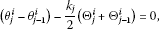(44)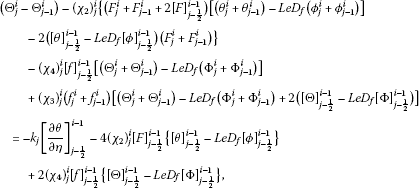(45)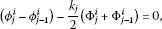(46)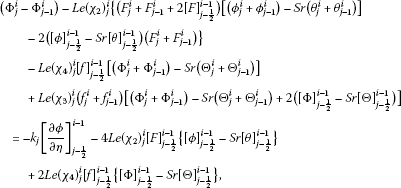(47)

where ${\zeta }_{j}^{i}=\frac{{k}_{j}}{2}\left[\frac{2}{3}{\eta }_{j-\frac{1}{2}}+\alpha \pi cos\left(\pi {\xi }_{i-\frac{1}{2}}-\phi \right){\xi }_{i-\frac{1}{2}}^{1/3}\right]$, ${\left({\chi }_{1}\right)}_{j}^{i}=\frac{{k}_{j}{\xi }_{i-\frac{1}{2}}}{{h}_{i}}$, ${\left({\chi }_{2}\right)}_{j}^{i}=\frac{\lambda {k}_{j}{\xi }_{i-\frac{1}{2}}}{4{h}_{i}}$, ${\left({\chi }_{3}\right)}_{j}^{i}=\frac{\lambda {k}_{j}}{4}\left(\frac{{\xi }_{i-\frac{1}{2}}}{{h}_{i}}+\frac{1}{6}\right)$ and ${\left({\chi }_{4}\right)}_{j}^{i}=\frac{\lambda {k}_{j}}{2}\left(\frac{{\xi }_{i-\frac{1}{2}}}{{h}_{i}}-\frac{1}{6}\right)$.

The boundary conditions take the form

${f}_{0}^{i}=0,\phantom{\rule{2em}{0ex}}{\theta }_{0}^{i}=0,\phantom{\rule{2em}{0ex}}{\varphi }_{0}^{i}=0,\phantom{\rule{2em}{0ex}}{F}_{J}^{i}=0,\phantom{\rule{2em}{0ex}}{\theta }_{J}^{i}=0,\phantom{\rule{2em}{0ex}}{\varphi }_{J}^{i}=0.$
(48)

The difference equations (42)-(47) are imposed at each grid points ${\eta }_{j}$, $j=1,2,\dots ,J$ giving rise to 6J algebraic equations. The boundary conditions (48) along with the difference equations (42)-(47) comprises a system of $6J+6$ equations for as many variables. There are several methods for solving these $6J+6$ equations. In this study, we employ Newton’s method which is easy to implement.

We use Newton’s method to linearize the nonlinear finite difference equations (42)-(47) which possesses quadratic nonlinearity. Assuming that the n th iterates of the variables are known the iterate ($n+1$)th iterates are given by

$\left[\begin{array}{c}{f}_{j}^{i,n+1}\\ {F}_{j}^{i,n+1}\\ {\theta }_{j}^{i,n+1}\\ {\mathrm{\Theta }}_{j}^{i,n+1}\\ {\varphi }^{i,n+1}\\ {\mathrm{\Phi }}_{j}^{i,n+1}\end{array}\right]=\left[\begin{array}{c}{f}_{j}^{i,n}\\ {F}_{j}^{i,n}\\ {\theta }_{j}^{i,n}\\ {\mathrm{\Theta }}_{j}^{i,n}\\ {\varphi }_{j}^{i,n}\\ {\mathrm{\Phi }}_{j}^{i,n}\end{array}\right]+\left[\begin{array}{c}\delta {f}_{j}^{i,n}\\ \delta {F}_{j}^{i,n}\\ \delta {\theta }_{j}^{i,n}\\ \delta {\mathrm{\Theta }}_{j}^{i,n}\\ \delta {\varphi }_{j}^{i,n}\\ \delta {\mathrm{\Phi }}_{j}^{i,n}\end{array}\right].$
(49)

We further define the following variables for brevity.

$\left[\begin{array}{c}{a}_{1j}\\ {a}_{2j}\\ {a}_{3j}\\ {a}_{4j}\\ {a}_{5j}\\ {a}_{6j}\end{array}\right]=\left[\begin{array}{c}{f}_{j}^{i,n}+{f}_{j-1}^{i,n}\\ {F}_{j}^{i,n}+{F}_{j-1}^{i,n}\\ {\theta }_{j}^{i,n}+{\theta }_{j-1}^{i,n}\\ {\mathrm{\Theta }}_{j}^{i,n}+{\mathrm{\Theta }}_{j-1}^{i,n}\\ {\varphi }_{j}^{i,n}+{\varphi }_{j-1}^{i,n}\\ {\mathrm{\Phi }}_{j}^{i,n}+{\varphi }_{j-1}^{i,n}\end{array}\right]\phantom{\rule{1em}{0ex}}\text{and}\phantom{\rule{1em}{0ex}}\left[\begin{array}{c}{b}_{1j}\\ {b}_{2j}\\ {b}_{3j}\\ {b}_{4j}\\ {b}_{5j}\\ {b}_{6j}\end{array}\right]=\left[\begin{array}{c}{f}_{j-1}^{i,n}-{f}_{j}^{i,n}\\ {F}_{j-1}^{i,n}-{F}_{j-1}^{i,n}\\ {\theta }_{j-1}^{i,n}-{\theta }_{j}^{i,n}\\ {\mathrm{\Theta }}_{j-1}^{i,n}-{\mathrm{\Theta }}_{j}^{i,n}\\ {\varphi }_{j-1}^{i,n}-{\varphi }_{j}^{i,n}\\ {\mathrm{\Phi }}_{j-1}^{i,n}-{\varphi }_{j}^{i,n}\end{array}\right].$
(50)

Substituting Eqs. (49)-(50) into Eqs. (42)-(47) and retaining only linear terms in δ, the linearized finite difference equations can be written as: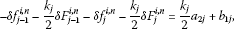(51)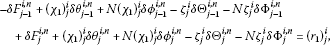(52)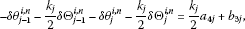(53)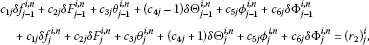(54)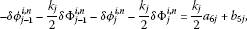(55)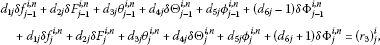(56)

where

$\begin{array}{c}{c}_{1j}={\left({\chi }_{3}\right)}_{j}^{i}\left\{{a}_{4j}-Le{D}_{f}{a}_{6j}+2\left({\left[\mathrm{\Theta }\right]}_{j-\frac{1}{2}}^{i-1}-Le{D}_{f}{\left[\mathrm{\Phi }\right]}_{j-\frac{1}{2}}^{i-1}\right)\right\},\hfill \\ {c}_{2j}=-{\left({\chi }_{2}\right)}_{j}^{i}\left\{{a}_{3j}-Le{D}_{f}{a}_{5j}-2\left({\left[\theta \right]}_{j-\frac{1}{2}}^{i-1}-Le{D}_{f}{\left[\varphi \right]}_{j-\frac{1}{2}}^{i-1}\right)\right\},\hfill \\ {c}_{3j}=-{\left({\chi }_{2}\right)}_{j}^{i}\left\{{a}_{2j}+2{\left[F\right]}_{j-\frac{1}{2}}^{i-1}\right\},\phantom{\rule{2em}{0ex}}{c}_{4j}={\left({\chi }_{3}\right)}_{j}^{i}{a}_{1j}-{\left({\chi }_{4}\right)}_{j}^{i}{\left[f\right]}_{j-\frac{1}{2}}^{i-1},\hfill \\ {c}_{5j}={\left({\chi }_{2}\right)}_{j}^{i}Le{D}_{f}\left\{{a}_{2j}+2{\left[F\right]}_{j-\frac{1}{2}}^{i-1}\right\},\phantom{\rule{2em}{0ex}}{c}_{6j}=-Le{D}_{f}\left\{{\left({\chi }_{3}\right)}_{j}^{i}{a}_{1j}-{\left({\chi }_{4}\right)}_{j}^{i}{\left[f\right]}_{j-\frac{1}{2}}^{i-1}\right\},\hfill \\ {d}_{1j}=\mathit{Le}{\left({\chi }_{3}\right)}_{j}^{i}\left\{{a}_{6j}-Sr{a}_{4j}+2\left({\left[\mathrm{\Phi }\right]}_{j-\frac{1}{2}}^{i-1}-Sr{\left[\mathrm{\Theta }\right]}_{j-\frac{1}{2}}^{i-1}\right)\right\},\hfill \\ {d}_{2j}=-\mathit{Le}{\left({\chi }_{2}\right)}_{j}^{i}\left\{{a}_{5j}-Sr{a}_{3j}-2\left({\left[\varphi \right]}_{j-\frac{1}{2}}^{i-1}-Sr{\left[\theta \right]}_{j-\frac{1}{2}}^{i-1}\right)\right\},\hfill \\ {d}_{3j}=LeSr{\left({\chi }_{2}\right)}_{j}^{i}\left\{{a}_{2j}+2{\left[F\right]}_{j-\frac{1}{2}}^{i-1}\right\},\phantom{\rule{2em}{0ex}}{d}_{4j}=-LeSr\left\{{\left({\chi }_{3}\right)}_{j}^{i}{a}_{1j}-{\left({\chi }_{4}\right)}_{j}^{i}{\left[f\right]}_{j-\frac{1}{2}}^{i-1}\right\},\hfill \\ {d}_{5j}=-\mathit{Le}{\left({\chi }_{2}\right)}_{j}^{i}\left\{{a}_{2j}+2{\left[F\right]}_{j-\frac{1}{2}}^{i-1}\right\},\phantom{\rule{2em}{0ex}}{d}_{6j}=\mathit{Le}\left\{{\left({\chi }_{3}\right)}_{j}^{i}{a}_{1j}-{\left({\chi }_{4}\right)}_{j}^{i}{\left[f\right]}_{j-\frac{1}{2}}^{i-1}\right\},\hfill \\ {\left({r}_{1}\right)}_{j}^{i}={b}_{2j}-{k}_{j}{\left[\frac{\partial F}{\partial \eta }\right]}_{j-\frac{1}{2}}^{i-1}+{\zeta }_{j}^{i}\left\{{a}_{4j}+N{a}_{6j}+2\left({\left[\mathrm{\Theta }\right]}_{j-\frac{1}{2}}^{i-1}+N{\left[\mathrm{\Phi }\right]}_{j-\frac{1}{2}}^{i-1}\right)\right\}\hfill \\ \phantom{{\left({r}_{1}\right)}_{j}^{i}=}-{\left({\chi }_{1}\right)}_{j}^{i}\left\{{a}_{3j}+N{a}_{5j}-2\left({\left[\theta \right]}_{j-\frac{1}{2}}^{i-1}+N{\left[\varphi \right]}_{j-\frac{1}{2}}^{i-1}\right)\right\},\hfill \\ {\left({r}_{2}\right)}_{j}^{i}={b}_{4j}-{k}_{j}{\left[\frac{\partial \mathrm{\Theta }}{\partial \eta }\right]}_{j-\frac{1}{2}}^{i-1}\hfill \\ \phantom{{\left({r}_{2}\right)}_{j}^{i}=}+{\left({\chi }_{2}\right)}_{j}^{i}\left\{{a}_{2j}+2{\left[F\right]}_{j-\frac{1}{2}}^{i-1}\right\}\left\{{a}_{3j}-Le{D}_{f}{a}_{5j}-2\left({\left[\theta \right]}_{j-\frac{1}{2}}^{i-1}-Le{D}_{f}{\left[\varphi \right]}_{j-\frac{1}{2}}^{i-1}\right)\right\}\hfill \\ \phantom{{\left({r}_{2}\right)}_{j}^{i}=}-\left\{{\left({\chi }_{3}\right)}_{j}^{i}{a}_{1j}-{\left({\chi }_{4}\right)}_{j}^{i}{\left[f\right]}_{j-\frac{1}{2}}^{i-1}\right\}\left\{{a}_{4j}-Le{D}_{f}{a}_{6j}+2\left({\left[\mathrm{\Theta }\right]}_{j-\frac{1}{2}}^{i-1}-Le{D}_{f}{\left[\mathrm{\Phi }\right]}_{j-\frac{1}{2}}^{i-1}\right)\right\},\hfill \\ {\left({r}_{3}\right)}_{j}^{i}={b}_{6j}-{k}_{j}{\left[\frac{\partial \mathrm{\Phi }}{\partial \eta }\right]}_{j-\frac{1}{2}}^{i-1}+\mathit{Le}{\left({\chi }_{2}\right)}_{j}^{i}\left\{{a}_{2j}+2{\left[F\right]}_{j-\frac{1}{2}}^{i-1}\right\}\left\{{a}_{5j}-Sr{a}_{3j}-2\left({\left[\varphi \right]}_{j-\frac{1}{2}}^{i-1}-Sr{\left[\theta \right]}_{j-\frac{1}{2}}^{i-1}\right)\right\}\hfill \\ \phantom{{\left({r}_{3}\right)}_{j}^{i}=}-\mathit{Le}\left\{{\left({\chi }_{3}\right)}_{j}^{i}{a}_{1j}-{\left({\chi }_{4}\right)}_{j}^{i}{\left[f\right]}_{j-\frac{1}{2}}^{i-1}\right\}\left\{{a}_{6j}-Sr{a}_{4j}+2\left({\left[\mathrm{\Phi }\right]}_{j-\frac{1}{2}}^{i-1}-Sr{\left[\mathrm{\Theta }\right]}_{j-\frac{1}{2}}^{i-1}\right)\right\}.\hfill \end{array}$

From the boundary conditions, we get the following difference equations:

$\delta {f}_{0}^{i,n}=0,\phantom{\rule{2em}{0ex}}\delta {\theta }_{0}^{i,n}=0,\phantom{\rule{2em}{0ex}}\delta {\varphi }_{0}^{i,n}=0,\phantom{\rule{2em}{0ex}}\delta {F}_{J}^{i,n}=0,\phantom{\rule{2em}{0ex}}\delta {\theta }_{J}^{i,n}=0,\phantom{\rule{2em}{0ex}}\delta {\varphi }_{J}^{i,n}=0.$
(57)

The linearized difference system of Eqs. (51)-(57) can be solved by any standard method like elimination or iterative methods. Since the linearized system has a block tridiagonal structure, we employ a block-elimination method. The block tridiagonal system in its matrix form is written as follows:

$\left[\begin{array}{cc}{A}_{0}& {C}_{0}\\ {B}_{1}& {A}_{1}& {C}_{1}\\ {B}_{2}& {A}_{2}& {C}_{2}\\ .& .& .\\ .& .& .\\ {B}_{J-1}& {A}_{J-1}& {C}_{J-1}\\ {B}_{J}& {A}_{J}\end{array}\right]\left[\begin{array}{c}{\mathrm{\Delta }}_{0}\\ {\mathrm{\Delta }}_{1}\\ {\mathrm{\Delta }}_{2}\\ .\\ .\\ {\mathrm{\Delta }}_{J-1}\\ {\mathrm{\Delta }}_{J}\end{array}\right]=\left[\begin{array}{c}{R}_{0}\\ {R}_{1}\\ {R}_{2}\\ .\\ .\\ {R}_{J-1}\\ {R}_{J}\end{array}\right],$
(58)

where

$\begin{array}{c}{A}_{0}=\left[\begin{array}{cccccc}1& 0& 0& 0& 0& 0\\ -1& -\frac{{k}_{1}}{2}& 0& 0& 0& 0\\ 0& 0& 1& 0& 0& 0\\ 0& 0& -1& -\frac{{k}_{1}}{2}& 0& 0\\ 0& 0& 0& 0& 1& 0\\ 0& 0& 0& 0& -1& -\frac{{k}_{1}}{2}\end{array}\right],\hfill \\ {A}_{J}=\left[\begin{array}{cccccc}0& 1& 0& 0& 0& 0\\ 0& 1& {\left({\chi }_{1}\right)}_{J}^{i}& -{\zeta }_{J}^{i}& N{\left({\chi }_{1}\right)}_{J}^{i}& -N{\zeta }_{J}^{i}\\ 0& 0& 1& 0& 0& 0\\ {c}_{1J}& {c}_{2J}& {c}_{3J}& {c}_{4J}+1& {c}_{5J}& {c}_{6J}\\ 0& 0& 0& 0& 1& 0\\ {d}_{1J}& {d}_{2J}& {d}_{3J}& {d}_{4J}& {d}_{5J}& {d}_{6J}+1\end{array}\right],\hfill \\ {A}_{j}=\left[\begin{array}{cccccc}-1& -\frac{{k}_{j}}{2}& 0& 0& 0& 0\\ 0& 1& {\left({\chi }_{1}\right)}_{j}^{i}& -{\zeta }_{j}^{i}& N{\left({\chi }_{1}\right)}_{j}^{i}& -N{\zeta }_{j}^{i}\\ 0& 0& -1& -\frac{{k}_{j}}{2}& 0& 0\\ {c}_{1j}& {c}_{2j}& {c}_{3j}& {c}_{4j}+1& {c}_{5j}& {c}_{6j}\\ 0& 0& 0& 0& -1& -\frac{{k}_{j}}{2}\\ {d}_{1j}& {d}_{2j}& {d}_{3j}& {d}_{4j}& {d}_{5j}& {d}_{6j}+1\end{array}\right],\phantom{\rule{1em}{0ex}}j=1,2,\dots ,J-1,\hfill \\ {B}_{j}=\left[\begin{array}{cccccc}0& 0& 0& 0& 0& 0\\ 0& -1& {\left({\chi }_{1}\right)}_{j}^{i}& -{\zeta }_{j}^{i}& N{\left({\chi }_{1}\right)}_{j}^{i}& -N{\zeta }_{j}^{i}\\ 0& 0& 0& 0& 0& 0\\ {c}_{1j}& {c}_{2j}& {c}_{3j}& {c}_{4j}-1& {c}_{5j}& {c}_{6j}\\ 0& 0& 0& 0& 0& 0\\ {d}_{1j}& {d}_{2j}& {d}_{3j}& {d}_{4j}& {d}_{5j}& {d}_{6j}-1\end{array}\right],\phantom{\rule{1em}{0ex}}j=1,2,\dots ,J,\hfill \\ {C}_{j}=\left[\begin{array}{cccccc}1& -\frac{{k}_{j}}{2}& 0& 0& 0& 0\\ 0& 0& 0& 0& 0& 0\\ 0& 0& 1& -\frac{{k}_{j}}{2}& 0& 0\\ 0& 0& 0& 0& 0& 0\\ 0& 0& 0& 0& 1& -\frac{{k}_{j}}{2}\\ 0& 0& 0& 0& 0& 0\end{array}\right],\phantom{\rule{1em}{0ex}}j=0,1,\dots ,J-1,\hfill \\ {R}_{0}=\left[\begin{array}{c}0\\ \frac{{k}_{1}}{2}{a}_{21}+{b}_{11}\\ 0\\ \frac{{k}_{1}}{2}{a}_{41}+{b}_{31}\\ 0\\ \frac{{k}_{1}}{2}{a}_{61}+{b}_{51}\end{array}\right],\phantom{\rule{2em}{0ex}}{R}_{J}=\left[\begin{array}{c}0\\ {\left({r}_{1}\right)}_{J}^{i}\\ 0\\ {\left({r}_{2}\right)}_{J}^{i}\\ 0\\ {\left({r}_{3}\right)}_{J}^{i}\end{array}\right],\phantom{\rule{2em}{0ex}}{R}_{j-1}=\left[\begin{array}{c}\frac{{k}_{j}}{2}{a}_{2j}+{b}_{1j}\\ {\left({r}_{1}\right)}_{j-1}^{i}\\ \frac{{k}_{j}}{2}{a}_{4j}+{b}_{3j}\\ {\left({r}_{2}\right)}_{j-1}^{i}\\ \frac{{k}_{j}}{2}{a}_{6j}+{b}_{5j}\\ {\left({r}_{3}\right)}_{j-1}^{i}\end{array}\right],\phantom{\rule{1em}{0ex}}j=2,3,\dots ,J,\hfill \\ {\mathrm{\Delta }}_{j}=\left[\begin{array}{c}\delta {f}_{j}^{i,n}\\ \delta {F}_{j}^{i,n}\\ \delta {\theta }_{j}^{i,n}\\ \delta {\mathrm{\Theta }}_{j}^{i,n}\\ \delta {\varphi }_{j}^{i,n}\\ \delta {\mathrm{\Phi }}_{j}^{i,n}\end{array}\right],\phantom{\rule{1em}{0ex}}j=0,1,\dots ,J.\hfill \end{array}$

We first factorize the coefficient matrix as follows:

$\begin{array}{c}\left[\begin{array}{cc}{A}_{0}& {C}_{0}\\ {B}_{1}& {A}_{1}& {C}_{1}\\ {B}_{2}& {A}_{2}& {C}_{2}\\ .& .& .\\ .& .& .\\ {B}_{J-1}& {A}_{J-1}& {C}_{J-1}\\ {B}_{J}& {A}_{J}\end{array}\right]\hfill \\ \phantom{\rule{1em}{0ex}}=\left[\begin{array}{c}{\mathrm{\Gamma }}_{0}\\ {B}_{1}& {\mathrm{\Gamma }}_{1}\\ {B}_{2}& {\mathrm{\Gamma }}_{2}\\ .& .\\ .& .\\ {B}_{J-1}& {\mathrm{\Gamma }}_{J-1}\\ {B}_{J}& {\mathrm{\Gamma }}_{J}\end{array}\right]\left[\begin{array}{cc}I& {\mathrm{\Lambda }}_{0}\\ I& {\mathrm{\Lambda }}_{1}\\ I& {\mathrm{\Lambda }}_{2}\\ .& .\\ .& .\\ I& {\mathrm{\Lambda }}_{J-1}\\ I\end{array}\right],\hfill \end{array}$

where I is an identity matrix of order 6. The procedure involves two sweeps, i.e., a forward sweep and a backward sweep. In the forward sweep, the unknowns ${\mathrm{\Gamma }}_{j}$, ${\mathrm{\Lambda }}_{j}$, and the intermediate variables ${\mathrm{\nabla }}_{j}$ are determined and in the backward sweep the required solution ${\mathrm{\Delta }}_{j}$ is determined in terms of ${\mathrm{\Lambda }}_{j}$ and ${\mathrm{\nabla }}_{j}$.

### Forward sweep

Initial matrices:

${\mathrm{\Gamma }}_{0}={A}_{0},\phantom{\rule{2em}{0ex}}{\mathrm{\Lambda }}_{0}={\mathrm{\Gamma }}_{0}^{-1}{C}_{0},\phantom{\rule{2em}{0ex}}{\mathrm{\nabla }}_{0}={\mathrm{\Gamma }}_{0}^{-1}{R}_{0}.$

Using the above, the successive matrices are obtained using the following formulae:

$\begin{array}{c}{\mathrm{\Gamma }}_{j}={A}_{j}-{B}_{j}{\mathrm{\Lambda }}_{j-1},\phantom{\rule{1em}{0ex}}j=1,2,\dots ,J,\hfill \\ {\mathrm{\Lambda }}_{j}={\mathrm{\Gamma }}_{j}^{-1}{C}_{j},\phantom{\rule{1em}{0ex}}j=1,2,\dots ,J-1,\hfill \\ {\mathrm{\nabla }}_{j}={\mathrm{\Gamma }}_{j}^{-1}\left({R}_{j}-{B}_{j}{\mathrm{\nabla }}_{j-1}\right),\phantom{\rule{1em}{0ex}}j=1,2,\dots ,J.\hfill \end{array}$

### Backward sweep

The required solution of the linearized system can be written as

$\begin{array}{c}{\mathrm{\Delta }}_{J}={\mathrm{\nabla }}_{J},\hfill \\ {\mathrm{\Delta }}_{j}={\mathrm{\nabla }}_{j}-{\mathrm{\Lambda }}_{j}{\mathrm{\Delta }}_{j+1},\phantom{\rule{1em}{0ex}}j=J-1,J-2,\dots ,0.\hfill \end{array}$

After obtaining the solution of the linearized system, the corrections are added to the solutions at the n th iteration and the procedure is repeated until convergence is achieved. This gives the solution at a given ${\xi }_{i}$. The procedure is to be repeated at the next streamwise location ${\xi }_{i+1}$ and so on. In boundary layer flows, the greatest error is associated with the wall gradients ${f}_{\eta }\left(\xi ,0\right)$, ${\theta }_{\eta }\left(\xi ,0\right)$, and ${\varphi }_{\eta }\left(\xi ,0\right)$, and hence these are used to determine convergence. The iterations are stopped when the successive iterate values of the gradients match up to 10−6. Since Newton’s method has second-order convergence, usually three to four iterations suffice to achieve the chosen tolerance.

## 4 Results and discussion

The problem of double diffusive convection induced by a horizontal wavy surface in a porous medium in presence of cross diffusion effect has been analyzed. The problem is governed by a system of coupled nonlinear parabolic partial differential equations (21)-(24). The Keller box method was used to find the numerical solutions of the problem. The amplitude of the wavy surface was assumed to be small and the wave phase was considered to be zero. The computations were carried out for the following range of parameter values; $0\le \alpha \le 0.5$, $-0.5\le N\le 1$, $0\le \mathit{Le}\le 5$ and $0\le {D}_{f}$, $\mathit{Le}\le 1$. We note here that $N>0$ represents the aiding buoyancy condition while $N<0$ represents the opposing buoyancy condition. The computations are shown in Figures 3-13. We mainly focus on the parametric effects on slip velocity and heat and mass transport coefficients.

Figure 3 shows the cross diffusion effects on the velocity, temperature, and concentration profiles for different values ξ. Due to the wavy nature of the surface, the dynamics of the fluid flow at different stream wise locations will be different. We notice the existence of locations where the velocity of the fluid attain extreme values over every undulation of the wavy surface. The profiles are shown for two selected stream wise locations, namely, $\xi =1$ and $\xi =5$. At both of these locations, the effect of cross diffusion is to increase both transverse and axial velocity profiles. The thermal and concentration boundary layers also thicken as a result of the cross diffusion phenomena as can be seen from Figures 3(c) and (d).

The variation of the slip velocity ${f}_{\eta }\left(\xi ,0\right)$ as a function of streamwise position ξ is shown for different wave amplitudes in Figure 4. For $\alpha =0$, the slip velocity remains constant indicating that self-similar solution exists in the plane surface case. For $\alpha \ne 0$, the slip velocity is oscillatory, increasing first to a maximum before decreasing to a minimum before the cycle is repeated. The fluctuations in the slip velocity increase with increasing amplitudes.

The heat transfer results are shown in Figures 5(a) and (b) for different values of the wave amplitude α. The local Nusselt number ${\mathit{Nu}}_{x}{\mathit{Ra}}^{-1/3}$ remains smooth and increases with ξ for the plane surface ($\alpha =0$). For the wavy surface ($\alpha \ne 0$), the local Nusselt number fluctuates over every cycle and these fluctuations rise as ξ increases. The fluctuations in the local Nusselt number increase with the wave amplitude. The mean Nusselt number ${\mathit{Nu}}_{m}{\mathit{Ra}}^{-1/3}$ increases with the stream position ξ and with increasing amplitude values.

Figures 5(c) and (d) highlight the mass transfer results for different values of α. Analogous to the heat transfer case, the local Sherwood number ${\mathit{Sh}}_{x}{\mathit{Ra}}^{-1/3}$ increases with ξ in the case of a plane surface ($\alpha =0$) while for a wavy surface ($\alpha \ne 0$) the local Sherwood number fluctuates. These fluctuations increase as ξ increases. Further, the fluctuations in the local Sherwood number increases with the wave amplitude. Figure 5(d) shows that the mean Sherwood number ${\mathit{Sh}}_{m}{\mathit{Ra}}^{-1/3}$ further increases with the stream position ξ and with increasing values of α. These results are qualitatively similar to those of Rees and Pop .

Figure 6 shows the effect of the Lewis number Le and the buoyancy ratio N on the slip velocity. The Lewis number reduces the slip velocity while the buoyancy ratio has an enhancing effect as expected. Increasing aiding buoyancy generates faster moving fluid flow as is evident in Figure 6(b).

Figure 7 shows the effect of buoyancy ratio N on the local and mean Nusselt and Sherwood numbers. It is evident that the buoyancy ratio enhances both the local and mean heat and mass transfer coefficients. The buoyancy ratio enhances the local and the mean Nusselt numbers. As observed earlier, ${\mathit{Nu}}_{x}{\mathit{Ra}}^{-1/3}$ and ${\mathit{Sh}}_{x}{\mathit{Ra}}^{-1/3}$ exhibits fluctuations which rise with the streamwise coordinate ξ. These results are in agreement with those reported by Cheng  with respect to a vertical wavy surface.

Figure 8 shows the effect of the Lewis number Le on the local and mean Nusselt and Sherwood numbers. The Lewis number tends to reduce the local and average heat transfer rates. Further, ${\mathit{Nu}}_{x}{\mathit{Ra}}^{-1/3}$ and ${\mathit{Sh}}_{x}{\mathit{Ra}}^{-1/3}$ exhibit fluctuations which increase with the streamwise coordinate ξ. These results regarding the heat and mass transfer coefficients coincide with those reported by Cheng  with respect to the vertical wavy surface. The effect of cross diffusion coefficients on the slip velocity is shown in Figure 9. The slip velocity ${f}_{\eta }\left(\xi ,0\right)$ is higher in the presence of cross diffusion effect as compared to the case when cross diffusion effect is absent.

The cross diffusion effect on the local and mean heat and mass transfer are depicted in Figure 10. These figures suggest that the presence of cross diffusion effects reduces both heat and mass transfer rates. The cross diffusion is more pronounced in case of heat transfer while the variation is infinitesimal in case of mass transfer. Figures 11(a) and (b) show the effect of the Dufour and Soret numbers on the slip velocity. Both parameters tend to accelerate the fluid in the boundary layer thereby increasing the slip velocity.

The effects of Dufour number on ${\mathit{Nu}}_{x}{\mathit{Ra}}^{-1/3}$, ${\mathit{Nu}}_{m}{\mathit{Ra}}^{-1/3}$, ${\mathit{Sh}}_{x}{\mathit{Ra}}^{-1/3}$ and ${\mathit{Sh}}_{m}{\mathit{Ra}}^{-1/3}$ is shown respectively in Figures 12(a)-(d). It is evident that the Dufour number reduces local and mean heat transfer rates while increasing the local and mean mass transfer rates. Here also we see the significant effect of ${D}_{f}$ in the case of heat transfer as compared to the mass transfer.

The effects of the Soret number on ${\mathit{Nu}}_{x}{\mathit{Ra}}^{-1/3}$, ${\mathit{Nu}}_{m}{\mathit{Ra}}^{-1/3}$, ${\mathit{Sh}}_{x}{\mathit{Ra}}^{-1/3}$, and ${\mathit{Sh}}_{m}{\mathit{Ra}}^{-1/3}$ are shown in Figures 13(a)-(d). These results show that the Soret number enhances the local and mean heat transfer rates while reducing the local and mean mass transfer rates. The effect of the Soret number is the exact opposite of that of the Dufour number on heat and mass transfer.

## 5 Conclusions

The paper analyzed double diffusive convection induced by a horizontal wavy surface embedded in a porous medium taking the cross diffusion effect into account. The governing parabolic partial differential equations have been solved using the Keller box method. The convergence rate depends on the parametric values chosen. Computations show that the Lewis number enhances mass transfer while reducing heat transfer. The buoyancy ratio enhances both the heat and mass transfer rates. The surface geometry also plays a crucial role in controlling heat and mass transfer rates. The effect of the Dufour number is to reduce heat transfer and enhance mass transfer. The effect of the Soret number is the exact opposite of the Dufour effect. We observe reduced heat and mass transfer in the presence of cross diffusion terms.

## References

1. Mojtabi A, Charrier-Mojtabi MC: Double diffusive convection in porous media. In Hand-book of Porous Media. Edited by: Vafai K. Taylor and Francis, New York; 2005:269-320.

2. Stern ME: The ‘salt fountain’ and thermohaline convection. Tellus 1960, 12: 172-175. 10.1111/j.2153-3490.1960.tb01295.x

3. Stern ME: Collective instability of salt fingers. J. Fluid Mech. 1969, 35: 209-218. 10.1017/S0022112069001066

4. Akbarzadeh A, Manins P: Convective layers generated by side walls in solar ponds. Sol. Energy 1988, 41(6):521-529. 10.1016/0038-092X(88)90055-2

5. Huppert HE, Sparks RSJ: Double-diffusive convection due to crystallization in magmas. Annu. Rev. Earth Planet. Sci. 1984, 12: 11-37. 10.1146/annurev.ea.12.050184.000303

6. Fernando HJS, Brandt A: Recent advances in double-diffusive convection. Appl. Mech. Rev. 1994, 47: c1-c7.

7. Ingham DB, Pop I: Transport Phenomena in Porous Media. Pergamon, Oxford; 1998.

8. Ingham DB, Pop I III. In Transport Phenomena in Porous Media. Elsevier, Oxford; 2005.

9. Nield DA, Bejan A: Convection in Porous Media. Springer, New York; 2006.

10. Vafai K: Handbook of Porous Media. Dekker, New York; 2000.

11. Vafai K: Handbook of Porous Media. Taylor and Francis, Boca Raton; 2005.

12. Vadasz P: Emerging Topics in Heat and Mass Transfer in Porous Media. Springer, New York; 2008.

13. Yao LS: Natural convection along a vertical wavy surface. J. Heat Transf. 1983, 105: 465-468. 10.1115/1.3245608

14. Moulic SG, Yao LS: Mixed convection along a wavy surface. J. Heat Transf. 1989, 111: 974-979. 10.1115/1.3250813

15. Moulic SG, Yao LS: Natural convection along a vertical wavy surface with uniform heat flux. J. Heat Transf. 1989, 111: 1106-1108. 10.1115/1.3250780

16. Rees DAS, Pop I: A note on free convection along a vertical sinusoidally wavy surface in a porous medium. J. Heat Transf. 1994, 116(1994):505-508.

17. Rees DAS, Pop I: Free convection induced by a vertical wavy surface with uniform heat flux in a porous medium. J. Heat Transf. 1995, 117: 545-550.

18. Rees DAS, Pop I: Free convection induced by a horizontal wavy surface in a porous medium. Fluid Dyn. Res. 1994, 14: 151-166. 10.1016/0169-5983(94)90026-4

19. Rees DAS, Pop I: The effect of longitudinal surface waves on free convection from vertical surfaces in porous media. Int. Commun. Heat Mass Transf. 1997, 24: 419-425. 10.1016/S0735-1933(97)00027-4

20. Cheng CY: Natural convection heat and mass transfer near a vertical wavy surface with constant wall temperature and concentration in a porous medium. Int. Commun. Heat Mass Transf. 2000, 27: 1143-1154. 10.1016/S0735-1933(00)00201-3

21. Cheng CY: Non-Darcy natural convection heat and mass transfer from a vertical wavy surface in saturated porous media. Appl. Math. Comput. 2006, 182: 1488-1500. 10.1016/j.amc.2006.05.037

22. Cheng CY: Combined heat and mass transfer in natural convection flow from a vertical wavy surface in a power-law fluid saturated porous medium with thermal and mass stratification. Int. Commun. Heat Mass Transf. 2009, 36: 351-356. 10.1016/j.icheatmasstransfer.2009.01.003

23. Cheng CY: Double diffusive natural convection along an inclined wavy surface in a porous medium. Int. Commun. Heat Mass Transf. 2010, 37: 1471-1476. 10.1016/j.icheatmasstransfer.2010.09.005

24. Pop I, Na TY: Natural convection of a frustum of wavy cone in a porous medium. Mech. Res. Commun. 1995, 22: 181-190. 10.1016/0093-6413(95)00010-0

25. Cheng CY: Natural convection heat and mass transfer near a wavy cone with constant wall temperature and concentration in a porous medium. Mech. Res. Commun. 2000, 27: 613-620. 10.1016/S0093-6413(00)00137-3

26. Cheng CY: Nonsimilar solutions for double diffusive convection near a frustum of a wavy cone in porous media. Appl. Math. Comput. 2007, 194: 156-167. 10.1016/j.amc.2007.04.074

27. Cheng CY: Double-diffusive natural convection along a vertical wavy truncated cone in non-Newtonian fluid saturated porous media with thermal and mass stratification. Int. Commun. Heat Mass Transf. 2008, 35: 985-990. 10.1016/j.icheatmasstransfer.2008.04.007

28. Mortimer RG, Eyring H: Elementary transition state theory of the Soret and Dufour effects. Proc. Natl. Acad. Sci. USA 1980, 77: 1728-1731. 10.1073/pnas.77.4.1728

29. Kafoussias NG, Williams EW: Thermal-diffusion and diffusion-thermo effects on mixed free-forced convective and mass transfer boundary layer flow with temperature dependent viscosity. Int. J. Eng. Sci. 1995, 33: 1369-1384. 10.1016/0020-7225(94)00132-4

30. Awad FG, Sibanda P, Motsa SS: On the linear stability analysis of a Maxwell fluid with double-diffusive convection. Appl. Math. Model. 2010, 34: 3509-3517. 10.1016/j.apm.2010.02.038

31. Onsager L: Reciprocal relations in irreversible processes-I. Phys. Rev. 1931, 37: 405-426. 10.1103/PhysRev.37.405

32. Alam MS, Rahman MM, Maleque MA, Ferdows M: Dufour and Soret effects on steady MHD combined free-forced convective and mass transfer flow past a semi-infinite vertical plate. Thammasat Int. J. Sci. Tech. 2006, 11: 1-12.

33. Shateyi S, Motsa SS, Sibanda P: The effects of thermal radiation, Hall currents, Soret, and Dufour on MHD flow by mixed convection over a vertical surface in porous media. Math. Probl. Eng. 2010., 2010: Article ID 627475. doi:10.1155/2010/627475

34. Narayana M, Sibanda P, Motsa SS, Lakshmi-Narayana PA: Linear and nonlinear stability analysis of binary Maxwell fluid convection in a porous medium. Heat Mass Transf. 2012, 48(5):863-874. 10.1007/s00231-011-0939-9

35. Malashetty MS, Biradar BS: The onset of double diffusive convection in a binary Maxwell fluid saturated porous layer with cross-diffusion effects. Phys. Fluids 2011., 23: Article ID 064102

36. Makinde OD: On MHD mixed convection with Soret and Dufour effects past a vertical plate embedded in a porous medium. Lat. Am. Appl. Res. 2011, 41: 63-68.

37. Makinde OD, Olanrewaju PO: Unsteady mixed convection with Soret and Dufour effects past a porous plate moving through a binary mixture of chemically reacting fluid. Chem. Eng. Commun. 2011, 198(7):920-938. 10.1080/00986445.2011.545296

38. Makinde OD: MHD mixed-convection interaction with thermal radiation and nth order chemical reaction past a vertical porous plate embedded in a porous medium. Chem. Eng. Commun. 2011, 198(4):590-608.

39. Keller HB: Numerical methods in boundary-layer theory. Annu. Rev. Fluid Mech. 1978, 10: 417-433. 10.1146/annurev.fl.10.010178.002221

40. Keller HB, Cebeci T: Accurate numerical methods for boundary layer flows 1: Two dimensional flows. Lecture Notes in Physics. In Proc. Int. Conf. Numerical Methods in Fluid Dynamics. Springer, Berlin; 1971.

## Acknowledgements

The authors are grateful to the National Research Foundation (NRF) and the University of KwaZulu-Natal for financial support.

## Author information

Authors

### Corresponding author

Correspondence to P Sibanda.

### Competing interests

The authors declare that they have no competing interests.

### Authors’ contributions

MN carried out the numerical computations and drafted the manuscript. PS, SSM, and PGS participated in the design of the study and helped to draft the manuscript. All authors read and approved the final manuscript.

## Authors’ original submitted files for images

Below are the links to the authors’ original submitted files for images.

## Rights and permissions

Reprints and Permissions

Narayana, M., Sibanda, P., Motsa, S. et al. On double-diffusive convection and cross diffusion effects on a horizontal wavy surface in a porous medium. Bound Value Probl 2012, 88 (2012). https://doi.org/10.1186/1687-2770-2012-88